# Practice with Lines

A Linear Relation can be expressed using the equation of a line:

$$y = mx + b$$

• $$y$$ is the dependent variable
• $$x$$ is the independent variable
• $$m$$ is the slope or rate of change
• $$b$$ is the y-intercept (the value of $$y$$ when $$x = 0$$)

In the diagram below, the line crosses the y-axis at $$y = 4$$. Every time $$x$$ increases by 1, $$y$$ increases by 2.

The equation of the given line is:

$$y = 2x + 4$$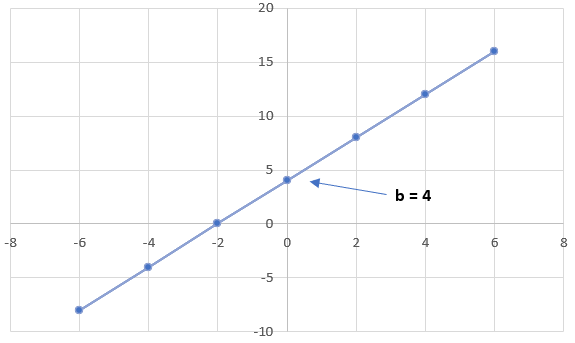When graphing a line with a given slope and y-intercept, you should ALWAYS begin at the y-intercept and move in the direction of the slope.

Write the equation of a line with a slope of 5 and a y-intercept of -3.

Rearrange the equation $$12x - 3y = 9$$ into slope-intercept form and state the values of m and b.

## Slope

You are able to calculate the Slope by calculating the Rise over the Run. The rise represents vertical distance between the two points. The run represents the horizontal distance between the two points. The slope can be determined algebraically as such:

$$m = \cfrac{\text{rise}}{\text{run}}\ = \cfrac{\Delta y}{\Delta x} = \cfrac{y₂-y₁}{x₂-x₁}$$

This formula calculates the change in $$y$$ (rise) divided by the change in $$x$$ (run) to measure the steepness (slope).

In order to determine the slope, select 2 separate points. Then plug the $$x$$ and $$y$$ values into the equation to calculate the slope. There are various different types of slopes depending on the resulting $$x$$ and $$y$$ values:

If $$m > 0$$, then the line will have a positive slope. This means that the two variables are positively related; that is, when $$x$$ increases, so does $$y$$, and when $$x$$ decreases, $$y$$ also decreases.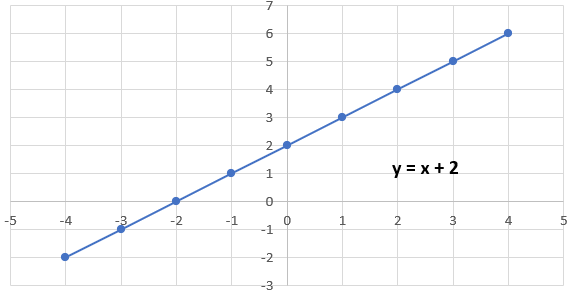If $$m < 0$$, then the line will have a negative slope. This means that the two variables are negatively related; that is, when $$x$$ increases, $$y$$ decreases, and when $$x$$ decreases, $$y$$ increases: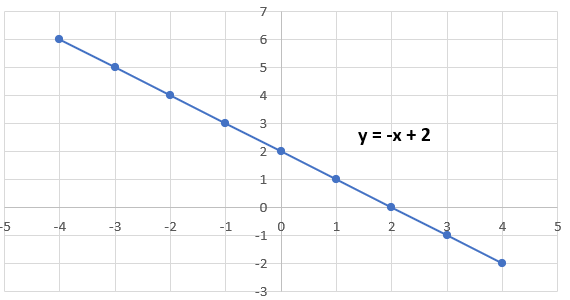If $$m = \cfrac{0}{x}$$, this will result in a slope of zero, resulting in a horizontal line. This means there is a constant relationship between $$x$$ and $$y$$; the $$y$$ value does not change when the value of $$x$$ changes. This can be expressed algebraically as $$y = b$$ where $$b$$ represents the y-int: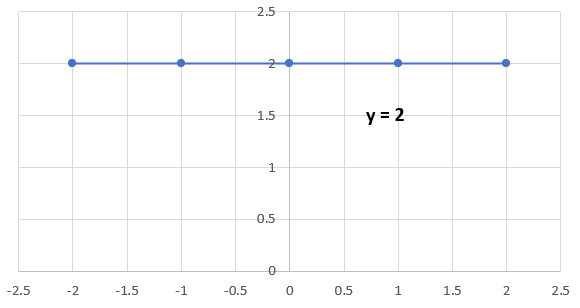If $$m = \cfrac{y}{0}$$, this will result, in an undefined slope, resulting in a vertical line. This means that the $$x$$ value does not change when the $$y$$ value changes. This can be expressed algebraically as $$x = a$$ where $$a$$ represents the x-int: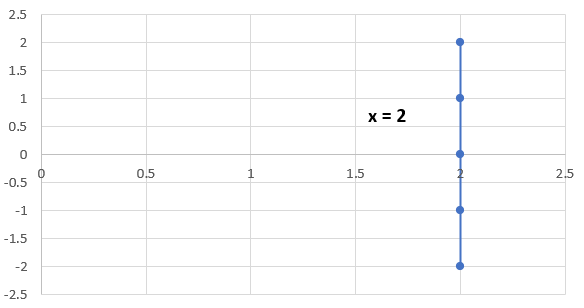Example

Determine the slope of a line with the points $$(4, -1)$$ and $$(6, 2)$$.

$$m = \cfrac{y₂-y₁}{x₂-x₁}$$

$$m = \cfrac{2-(-1)}{6-4}$$

$$m = \cfrac{3}{2} = 1.5$$

Thus, the slope of the line is $$1.5$$.

Fill in the next 3 sets of values in the given table of values.
 x y 6 4 2 -5 -2 1

## Intercepts

An intercept is a point where the line crosses an axis.

• An x-intercept is the point where the line crosses the x-axis
• A y-intercept is the point where the line crosses the y-axis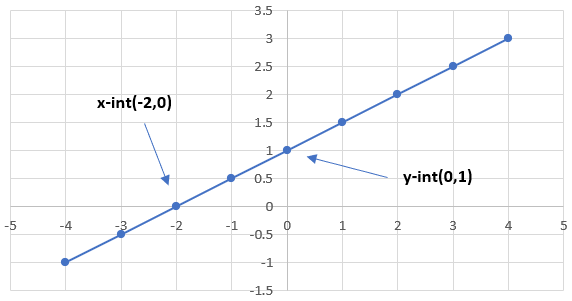Notice that the x-value of the y-int and the y-value of the x-int are 0. In order to solve for the x-intercept or the y-intercept, we must set the opposite value to $$0$$.

## Process for Solving x-Intercepts

1. Arrange the equation into the form $$y = mx + b$$
2. If the coefficient of $$y$$ (or $$a$$ ≠ $$1$$, divide the entire equation by $$a$$
3. Set $$y = 0$$
4. Move the variables and constants onto separate sides
5. Divide both sides by the coefficient of $$x$$ (or $$b$$) to solve the x-intercept

## Process for Solving y-Intercepts

1. Arrange the equation into the form $$y = mx + b$$
2. Set $$x = 0$$
3. Divide both sides by the coefficient of y (or a) to solve the y-intercept

Example

Find the intercepts for the equation $$y = 2x + 3$$

To find the x-intercept, set y = 0. Then, solve for $$x$$:

$$0 = 2x + 3$$
$$\cfrac{-2x}{\textcolor{red}{-2}} = \cfrac{3}{\textcolor{red}{-2}}$$

$$x = -\cfrac{3}{2}$$

Therefore, we can determine that the the x-intercept is $$(-3/2,0)$$.

To find the y-intercept, set $$x = 0$$. Then, solve for $$y$$:

$$y = 2(0) + 3$$
$$y = 3$$

Therefore, we can determine that the y-intercept is $$(0,3)$$.

Find the intercepts for the equation $$–10y = -5x + 120$$